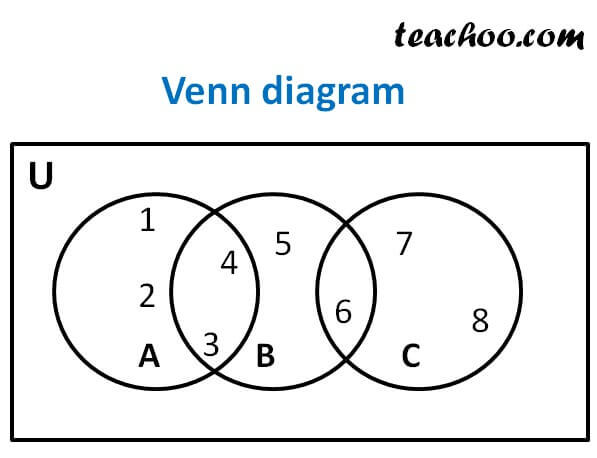Venn Diagram and Union of Set

Chapter 1 Class 11 Sets (Term 1)
Concept wise

Venn diagram is the representation of sets using circles.

Let us take sets

Let A = {1, 2, 3, 4} , B = {3, 4, 5, 6}, C = {6, 7, 8}

and Universal set = U = {1, 2, 3, 4, 5, 6, 7, 8, 9, 10}

Then, the Venn Diagram will be